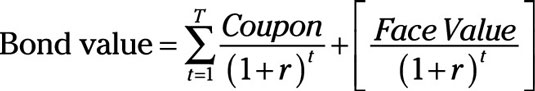##### Corporate Finance For Dummies, 2nd EditionThe valuation of bonds refers to the process by which you determine the value of a bond. This information is then used, in conjunction with your personal estimates of what you’re willing to pay or your other options, to determine what is considered a fair price.

For investors, these valuation methods are the manner in which the investor will figure out what they’re willing to pay, what they can expect in returns, and what their investment portfolio is worth at any given point in time.

For the issuing organizations, these valuation methods allow them to determine how much capital they can raise using debt, and the interest rates they will have to offer in order to attract investors. You will do this using math!r stands for “rate”, which is the annual interest rate, and t is the number of years that the single cash flow, so all this equation says is that you need to add up the present values of all the coupons, then also add the present value of the end principal payment, and that gives you the total present value of the bond.

That thing at the front that looks like a giant “E” is called a sigma. All it means is that you’re adding the values of different things together; in this case, the different present values of future coupon cash flows for each year.

The comforting part of understanding this equation is that even the more complicated equations are really just variations that build on this same theme using additional variables and information to refine it and make it more accurate.

Zero-coupon bonds, principal STRIPS, and other bonds that don’t make periodic interest payments don’t have this sort of calculation. Instead, because they generate all their cash flows at maturity, the bond value is equal to the present value of the single future cash flow after accounting for accumulated interest. Just look at them with the present value of its maturity date, rather than including any coupon payments.

If you’re not holding a bond to maturity, or you want to calculate your percentage return on bond investment, you can do so by calculating the holding period yield. This is the amount of yield that a bond will provide while a person is holding it, which pretty much assumes that the person is selling the bond before maturity — but some people just really like math.

Holding period yield = {[Coupon + (Net gain/Loss)]/Purchase price} x 100

In this case, the net gain or loss is the price of selling the bond minus the price of purchasing the bond, meaning that it’s the profits generated from buying and reselling the bond. Add any coupon payments you received during the holding period, and you’ve just calculated what the bond is worth over a holding period rather than to maturity.

Of course, this does assume you can accurately estimate the price you can sell the bond for, which is a pretty big assumption for some people. Take the value of that bond and divide it by your purchase price to show your return on investment as a proportion; multiply it by 100 to calculate it as a percentage.